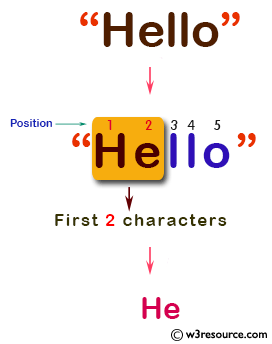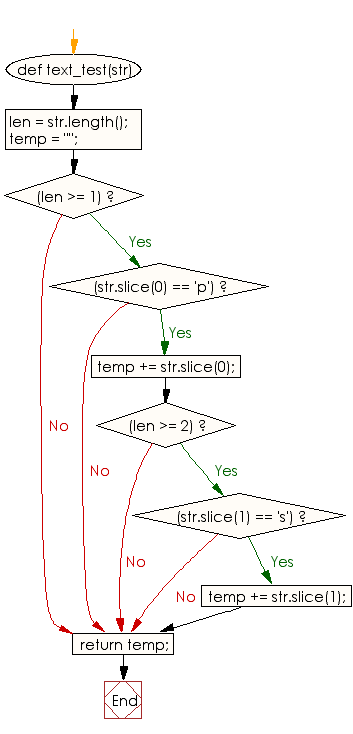﻿ Ruby Basic exercises: Create a string using the first two characters - w3resource# Ruby Basic Exercises: Create a string using the first two characters

## Ruby Basic: Exercise-35 with Solution

Write a Ruby program to create a string using the first two characters (if present) of a given string if the first character is 'p' and second one is 's' otherwise return a blank string.Ruby Code:

``````def text_test(str)
len = str.length();
temp = "";
if(len >= 1)
if(str.slice(0) == 'p')
temp += str.slice(0);
if(len >= 2)
if(str.slice(1) == 's')
temp += str.slice(1);
end
end
end
end
return temp;
end
print text_test("psabcd"),"\n"
print text_test("abcd")
``````

Output:

```ps
```

Flowchart:Ruby Code Editor: